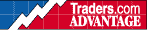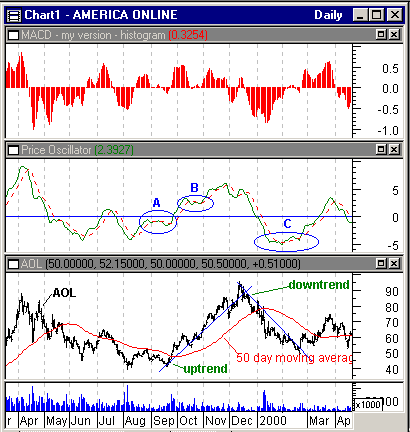HOT TOPICS LIST

INDICATORS LIST

LIST OF TOPICS

# MACD (Part I Of II)

05/07/01 04:25:40 PM
by Dennis D. Peterson

Analyzing how MACD works makes a good case study for many indicators.

Security:   AOL
Position:   N/A

 MACD has two components. The first component is the difference of two moving averages. The second component is called the signal line and is the moving average of the difference of the moving averages. Create MACD by creating the price oscillator, PO, and then the moving average of PO. The price oscillator is the difference of two moving averages. Moving averages are trend-following devices. If a stock or commodity is in a trend, then MACD should work well. From previous postings you know that I would recommend swing points as an alternative for trend trading, but there is much to be learned from MACD. MACD is especially attractive in the currency commodity markets, since currencies can have longer-term trends. Some analysts, names you know, would even argue that using a trend following device in the stock market is a highly questionable endeavor. That's why you see many examples of MACD using commodities, such as currencies. In order to analyze MACD behavior I am going to use its two components, PO and the moving average of PO to avoid any confusion. The default for MACD is a 26-day moving average subtracted from a 12-day moving average, and a nine-day moving average of the difference for the signal line. If I chart PO as the point difference of a 12-day and 26-day moving average and overlay PO on MACD, I completely cover MACD except the signal line. If I then take a nine-day moving average of PO, I have a signal line that is exactly the same as the MACD signal line. PO is a momentum indicator because it compares more current prices (by using the shorter period moving average) with those in the past (by using the longer period moving average). PO, and therefore MACD, measures the strength or enthusiasm of the trend. As a difference of two moving averages, a plot of PO will become horizontal when the difference is constant. A constant difference means that (averaged) new prices are changing at the same rate as (averaged) old prices. The consequence is that when PO goes flat (horizontal), the moving average of PO can easily move above or below PO: especially if the moving average of PO has a short period. If your trading system uses the crossing of PO with its average, this means you are likely to be in and out frequently -- whipsawed.In Figure 1 (middle chart) the flat periods in momentum are shown as areas A, B, and C. The potential of getting whipsawed is high because the moving average of PO, the signal line, can easily cross PO. Areas B and C both occur while AOL is in a trend -- a little discouraging, since you might reasonably expect that a strong price trend should produce unequivocal momentum. You might now notice that if PO is positive it doesn't even guarantee that you are in an uptrend. Before it gets better, you need to make a few more observations. Figure 1 also shows the difference between a simple moving average, of 50 days for example, and the momentum of moving averages. On the bottom chart I have overlaid the daily AOL price data with a 50-day simple moving average and the middle chart is PO and the moving average of PO, i.e. MACD. If you were to trade the 50-day moving average by going long when price is above the moving average and short when price is below the moving average you would have made money (\$1,000 invested in '96 would be \$5,000 today). You also would have made money going short when PO is below its moving average and going long when PO is above its moving average (\$1,000 invested in '96 would be \$3,000 today). What's a bit discouraging is that you would have made more using only a simple moving average.Figure 1: Top chart is MACD histogram (difference between PO and the moving average of PO). Middle chart is PO and the moving average of PO (same as MACD). Bottom chart is AOL daily price and volume history with a 50-day moving average (red) and two trends identified. Graphic provided by: MetaStock. Graphic provided by: Data vendor: eSignal<. The other side of the coin of a constant rate of price change producing flat momentum, and the potential of being whipsawed, is the behavior of momentum when rates are changing. You will notice that the difference between PO and the moving averages varies. Plotting the difference between PO and the moving average of PO generates the top chart of Figure 1. The MACD histogram!The histogram is the visual representation of how much the momentum is above or below its own moving average. It is no accident that when price is furthest away from the trendlines, the histogram shows the greatest values. The histogram is MACD momentum. That's right, the momentum of the momentum. Interpreting a trendline gives you much of the same information. The book "Technical Analysis of Stock Trends" by Robert Edwards and John Magee is worth reading - over and over again. It reminds me of someone that I knew and as I got older they got wiser. The more you understand the more Edwards and Magee gets better. MACD is a convenience with some encumbrances, but using the momentum of MACD allows a certain degree of autopilot.For Part II I will show equity performance for three trading systems and explain the performance in terms of trend behavior and MACD.

Dennis D. Peterson

Market index trading on a daily basis.

 Comments or Questions? Article Usefulness 5 (most useful) 4 3 2 1 (least useful)

Date:�05/15/01Rank:�4Comment:
Date:�04/16/02Rank:�4Comment:
Date:�07/01/03Rank:�5Comment: